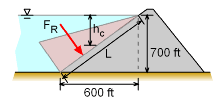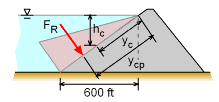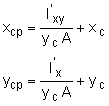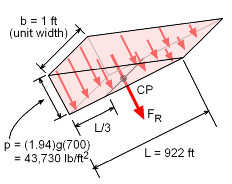Ch 2. Fluid Statics Multimedia Engineering Fluids PressureVariation PressureMeasurement HydrostaticForce(Plane) HydrostaticForce(Curved) Buoyancy
 Chapter 1. Basics 2. Fluid Statics 3. Kinematics 4. Laws (Integral) 5. Laws (Diff.) 6. Modeling/Similitude 7. Inviscid 8. Viscous 9. External Flow 10. Open-Channel Appendix Basic Math Units Basic Equations Water/Air Tables Sections Search eBooks Dynamics Fluids Math Mechanics Statics Thermodynamics Author(s): Chean Chin Ngo Kurt Gramoll ©Kurt GramollFLUID MECHANICS - CASE STUDY SOLUTIONProblem Diagram I The magnitude of the resultant force is given by       FR = ρghcA The vertical distance to the centroid of the dam surface is given by      hc = 700 / 2 = 350 ft The total length L of the dam surface is calculated to be      L = (6002 + 7002)0.5 = 922 ftProblem Diagram II The magnitude of the resultant force is thus given by      FR = (1.94)(32.2)(350)(922) = 20.2 (106) lb The coordinates for the center of pressure are given byFrom the appendix, for a rectangle,      Ix' = (922)3(1) / 12      Ixy' = 0 Hence, it is found that      xcp = xc which is at the centerline of the dam surface. The y coordinate for the center of pressure isPressure Prism ycp = (922)3(1) / (12)(461)(922) + 461 = 614.7 ft The pressure prism approach can be used to verify the results obtained. Based on the pressure prism shown in the figure, the magnitude of the resultant force is represented by the total volume of the pressure prism.      FR = Volume = 1/2 (43,730)(1)(922) = 20.2 (106) lb This resultant force passes through the centroid of the pressure prism, which is located at a distance of 922/3 = 307.3 ft from its base and lies in the vertical symmetry axis.

Practice Homework and Test problems now available in the 'Eng Fluids' mobile app
Includes over 250 problems with complete detailed solutions.
Available now at the Google Play Store and Apple App Store.